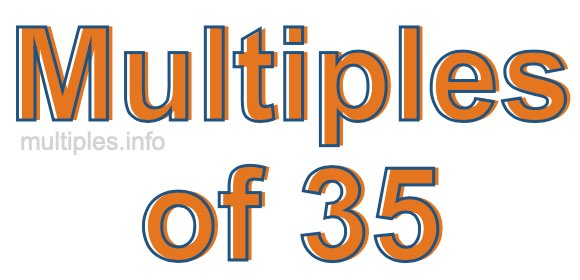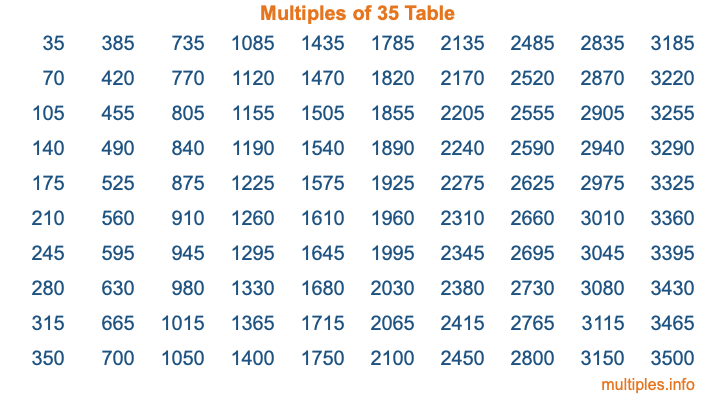Multiples of 35Welcome to the Multiples of 35 page. Here we will first teach you everything you will ever need to know about the multiples of 35, and then give you a study guide summary of everything we taught you to make sure you remember it all. Use this page to look up facts and learn information about the multiples of 35. This page will make you a multiples of thirty-five expert!

Definition of Multiples of 35
Multiples of 35 are all the numbers that when divided by 35 equal an integer. Each of the multiples of 35 are called a multiple. A multiple of 35 is created by multiplying 35 by an integer.

Therefore, to create a list of multiples of 35, you start with 1 multiplied by 35, then 2 multiplied by 35, then 3 multiplied by 35, and so on for as long as you want. Thus, the list of the first five multiples of 35 is 35, 70, 105, 140, and 175. To see a larger list of multiples of 35, see the printable image of Multiples of 35 further down on this page. We also have a category where you can choose any nth multiple of 35.

Multiples of 35 Checker
The Multiples of 35 Checker below checks to see if any number of your choice is a multiple of 35. In other words, it checks to see if there is any number (integer) that when multiplied by 35 will equal your number. To do that, we divide your number by 35. If the the quotient is an integer, then your number is a multiple of 35.

Is  a multiple of 35?

Least Common Multiple of 35 and ...
A Least Common Multiple (LCM) is the lowest multiple that two or more numbers have in common. This is also called the smallest common multiple or lowest common multiple and is useful to know when you are adding our subtracting fractions. Enter one or more numbers below (35 is already entered) to find the LCM.

Check out our LCM Calculator if you need more details about the Least Common Multiple or if you need the LCM for different numbers for adding and subtraction fractions.

nth Multiple of 35
As we stated above, 35 is the first multiple of 35, 70 is the second multiple of 35, 105 is the third multiple of 35, and so on. Enter a number below to find the nth multiple of 35.

th multiple of 35

Multiples of 35 vs Factors of 35
35 is a multiple of 35 and a factor of 35, but that is where the similarities end. All postive multiples of 35 are 35 or greater than 35. All positive factors of 35 are 35 or less than 35.

Below is the beginning list of multiples of 35 and the factors of 35 so you can compare:

Multiples of 35: 35, 70, 105, 140, 175, etc.

Factors of 35: 1, 5, 7, 35

As you can see, the multiples of 35 are all the numbers that you can divide by 35 to get a whole number. The factors of 35, on the other hand, are all the whole numbers that you can multiply by another whole number to get 35.

It's also interesting to note that if a number (x) is a factor of 35, then 35 will also be a multiple of that number (x).

Multiples of 35 vs Divisors of 35
The divisors of 35 are all the integers that 35 can be divided by evenly. Below is a list of the divisors of 35.

Divisors of 35: 1, 5, 7, 35

The interesting thing to note here is that if you take any multiple of 35 and divide it by a divisor of 35, you will see that the quotient is an integer.

Multiples of 35 Table
Below is an image of the first 100 multiples of 35 in a table. The table is in chronological order, column by column. The first column has the first ten multiples of 35, the second column has the next ten multiples of 35, and so on.The Multiples of 35 Table is also referred to as the 35 Times Table or Times Table of 35. You are welcome to print out our table for your studies.

Negative Multiples of 35
Although not often discussed or needed in math, it is worth mentioning that you can make a list of negative multiples of 35 by multiplying 35 by -1, then by -2, then by -3, and so on, to get the following list of negative multiples of 35:

-35, -70, -105, -140, -175, etc.

Multiples of 35 Summary
Below is a summary of important Multiples of 35 facts that we have discussed on this page. To retain the knowledge on this page, we recommend that you read through the summary and explain to yourself or a study partner why they hold true.

There are an infinite number of multiples of 35.

A multiple of 35 divided by 35 will equal a whole number.

35 divided by a factor of 35 equals a divisor of 35.

The nth multiple of 35 is n times 35.

The largest factor of 35 is equal to the first positive multiple of 35.

35 is a multiple of every factor of 35.

35 is a multiple of 35.

A multiple of 35 divided by a divisor of 35 equals an integer.

35 divided by a divisor of 35 equals a factor of 35.

Any integer times 35 will equal a multiple of 35.

Multiples of a Number
Here you can get the multiples of another number, all with the same attention to detail as we did for multiples of 35 on this page.

Multiples of
Multiples of 36
Did you find our page about multiples of thirty-five educational? Do you want more knowledge? Check out the multiples of the next number on our list!